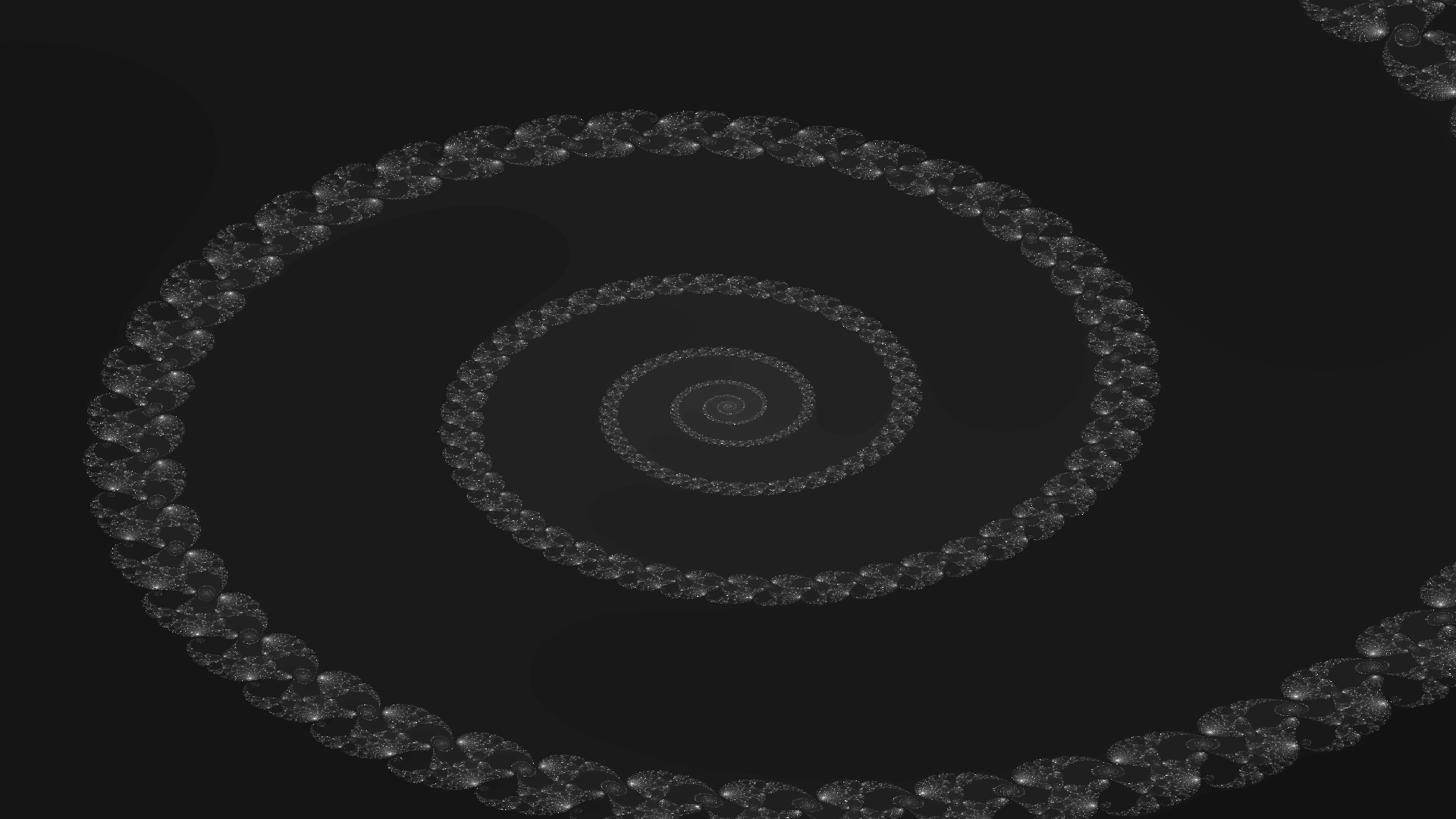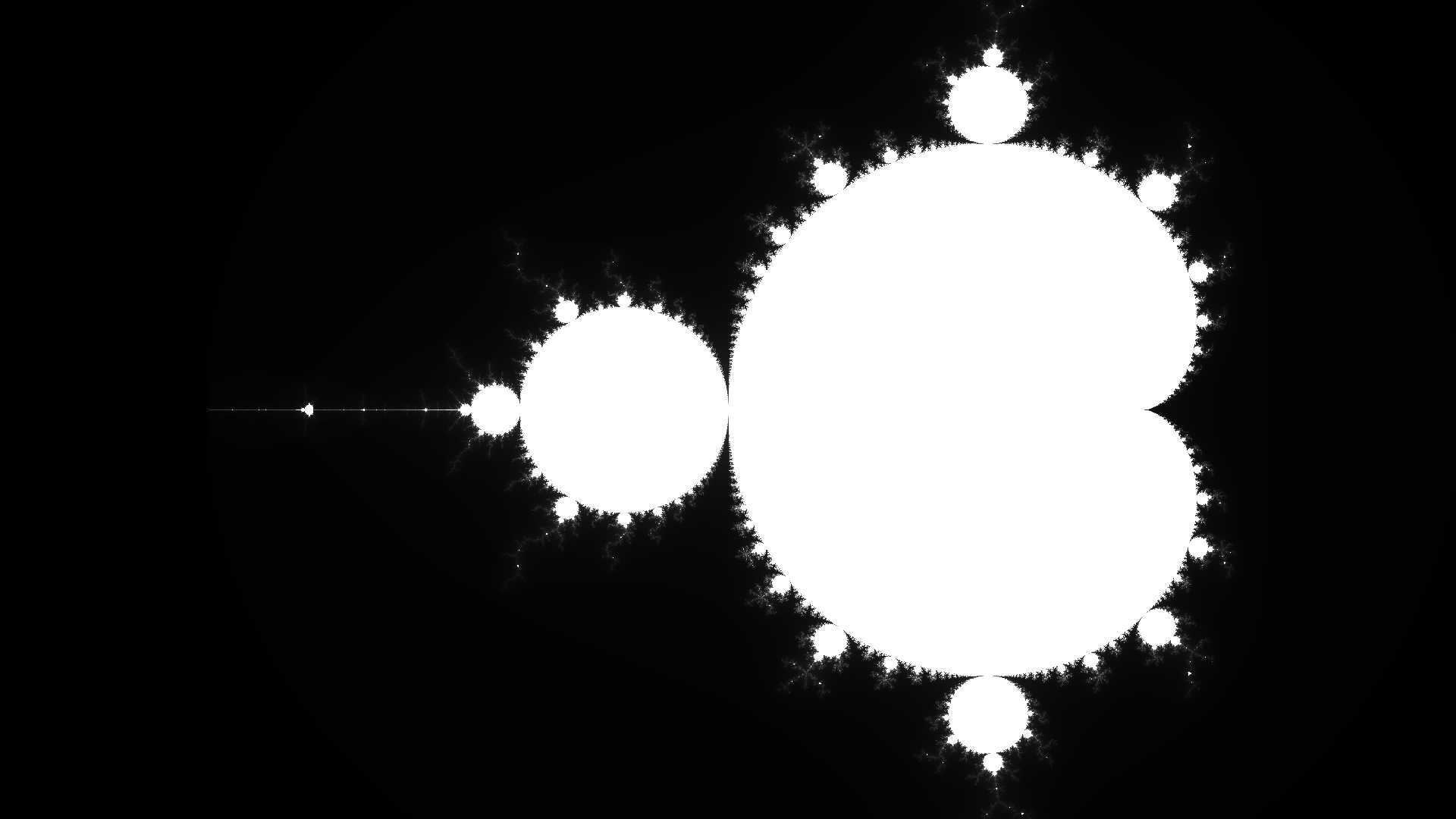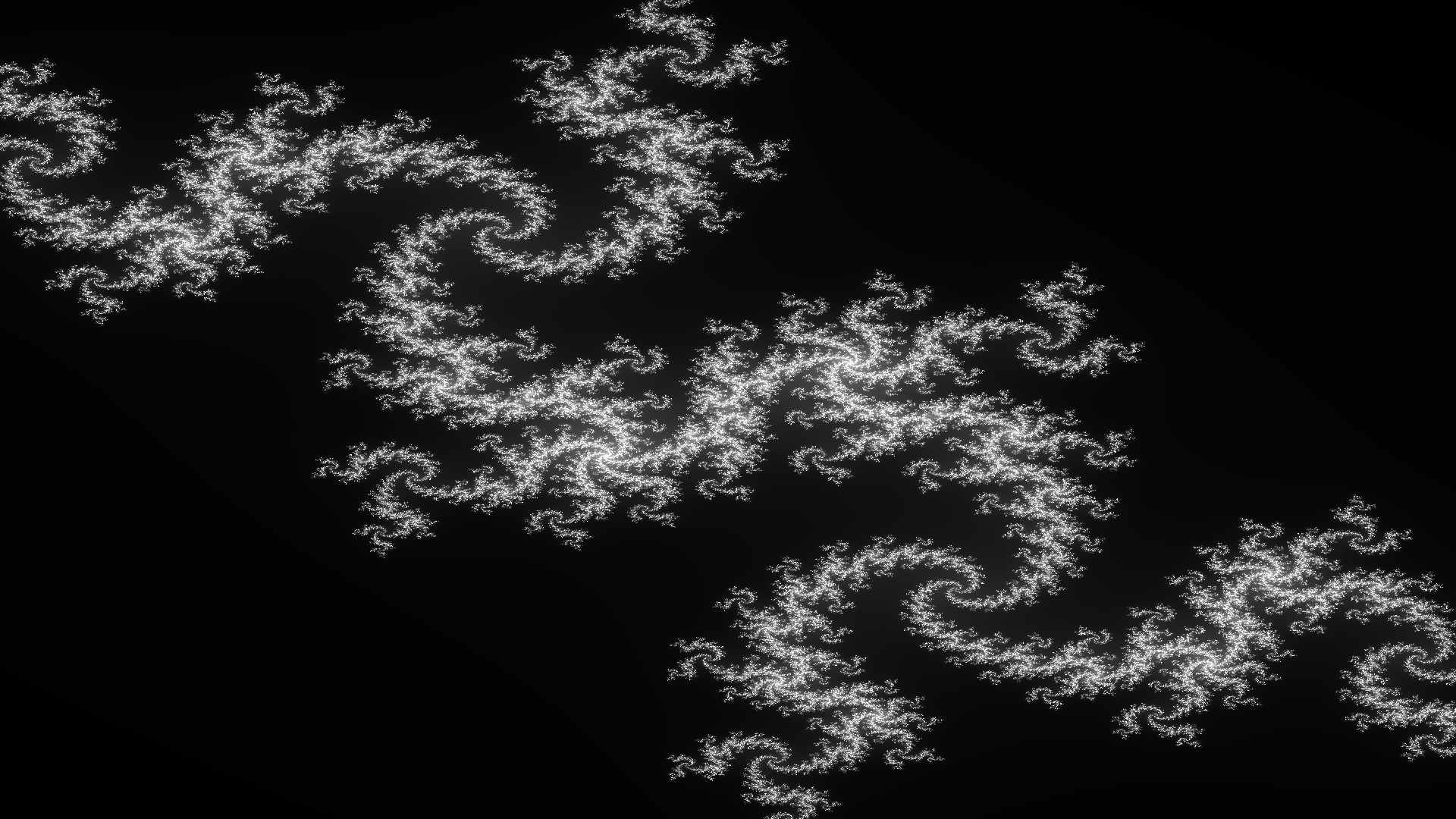# Julia Set

Topic: Challenges, Date: 01/08/2016

## Description

This challenge was picked from the r/dailyprogrammer subreddit. The challenge is to generate Julia set fractals from the complex number plane and produce an image as the output. This was a great chance to use Go as one of the bonus challenges is to make the solution run in parallel. This meant that using goroutines was an easy way to solve what could have been a much more difficult challenge.

I added a few options to the package so that the user can change the function that decides the Julia set (which also happened to be a bonus round). This is done by calling the SetComplexFunction function and supplying it with a function that has the signature of func(complex128, complex128) complex128 . The first parameter is the current complex number on this iteration, and the second is the original complex number for the pixel value. This second parameter allows the generation of the basic Mandelbrot set. I've also added the option to change the complex number range, this allows me to zoom in or out of a particularly interesting set (I use this to view the whole Mandelbrot set).

## Mandelbrot Set

Even though this package was mostly built for the creation of Julia set images, you can also display the Mandelbrot set and other complex fractals by using the SetComplexFunction . Mandelbrot is defined by the function $f_c(z) = z^2 + c$ where $c$ is the complex number and $z$ is the iteration. This is more explicit when represented recursively, $z_{n} = z^2_{n-1} + c$. The code snippet below shows how to draw the Mandelbrot set using this package.

julia.SetComplexFunction(func(c, orig complex128) complex128 {
return c*c + orig
})
julia.SetComplexRange(complex(-2.5, -1), complex(1, 1))
julia.CreateImage("image.png", 1920, 1080)

## Zooming Into A Fractal

As previously mentioned you can zoom into a fractal by using the SetComplexZoom and SetMaxIteration functions. We can also alter the contrast of the image using the SetContrast function. This will help to show the patterns more clearly.

The snippet below shows how to zoom into the Mandelbrot set. For other interesting coordinates, you can look at http://www.cuug.ab.ca/dewara/mandelbrot/images.html.

julia.SetComplexFunction(func(c, orig complex128) complex128 {
return c*c + orig
})
julia.SetColorFunction(func(col uint8) *color.RGBA {
return &color.RGBA{col, col, col, 255}
})
julia.SetComplexZoom(complex(0.2549870375144766, -0.0005679790528465), 1.0e-13)
julia.SetContrastValue(50.0)
julia.SetMaxIteration(5000)
julia.CreateImage("image.png", 1920, 1080)

## Feedback From A Friendly Redditor